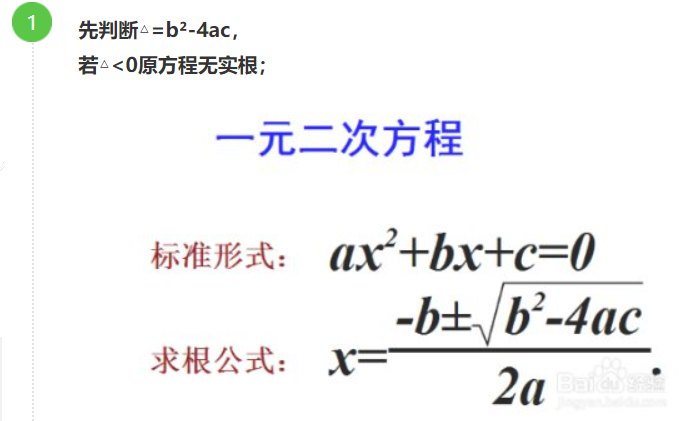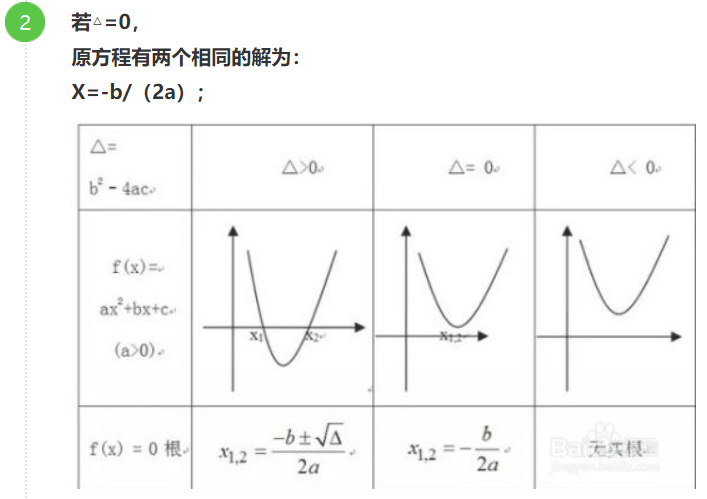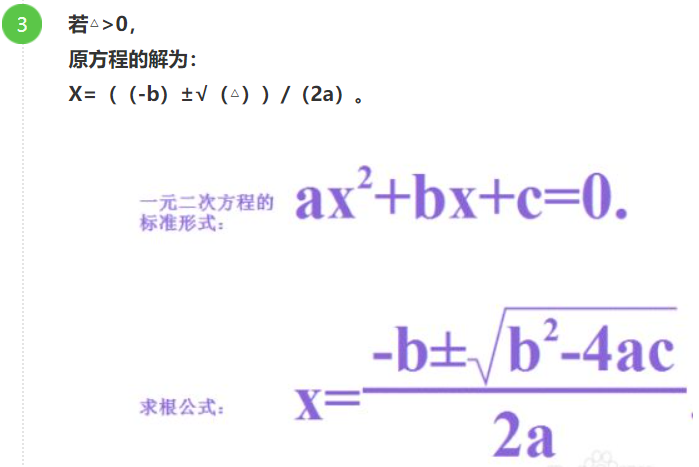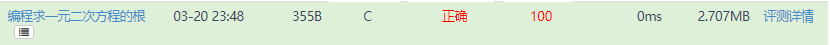• 编程求一元二次方程
千次阅读
2017-10-06 18:23:43
#define EXP 0.00000001
int main()
{
float a = 0.0, b = 0.0, c = 0.0;
scanf("%f%f%f", &a, &b, &c);
float disc = b*b - 4 * a*c;
if ((a>-EXP) && (a<EXP))
{
printf("不是一元二次方程！\n");
}
else
{
if (disc > -EXP && disc < EXP)
{
printf("有相同解%f\n", -b / (2 * a));
}
else if (disc>0.0)
{
printf("方程有俩解%f，%f\n", (-b + sqrt(disc)) / (2 * a), (-b - sqrt(disc)) / (2 * a));
}
else
{
printf("方程无解！\n");
}
}
return 0;

}C语言
更多相关内容
• 主要介绍了Python编程实现数学运算求一元二次方程的实根算法,涉及Python数学运算求解方程的相关实现技巧,需要的朋友可以参考下
• 　编写一个求解一元二次方程的实数根的程序，方程的系数由用户在运行xh 输入格式 　输入一行三个整数分别为一元二次方程的三个系数，数据之间以空格隔开 输出格式 　输出一行为方程的实数根（若两根不同 较大的在前 ...
问题描述
编写一个求解一元二次方程的实数根的程序，方程的系数由用户在运行xh
输入格式
输入一行三个整数分别为一元二次方程的三个系数，数据之间以空格隔开
输出格式
输出一行为方程的实数根（若两根不同 较大的在前 两根以空格隔开 若两根相同 输出一个 若无根 输出 NO ）
样例输入
1 -5 4
样例输出
4 1
样例输入
1 -2 1
样例输出
1
样例输入
1 0 1
样例输出
NO


题解思路：

公式法：代码如下：

#include<stdio.h>
#include<math.h>
int main() {
double a,b,c;
scanf("%lf%lf%lf",&a,&b,&c);
double root=0,x1=0,x2=0;
root=b*b-4*a*c;
if(root>0){
x1=(-b+sqrt(root))/(2*a);
x2=(-b-sqrt(root))/(2*a);
if(x1>x2){
printf("%g %g",x1,x2);
}
}else if(root==0){
x1=(-b)/(2*a);
x2=x1;
printf("%g",x1);
}else{
printf("NO");
}

return 0;
}本题要注意一个精度输出，不然难过一些事数据。比如2 5 1.

printf("%g %g",x1,x2);


此行代码中%g是什么意思。
%g是C语言printf()函数的一个输出格式类型，它表示以%f%e中较短的输出宽度输出单、双精度实数，在指数小于-4或者大于等于精度时使用%e格式。

举个例子

printf("%g\n", 0.00001234);

printf("%g\n", 0.0001234);

printf("%.2g\n", 123.45);

printf("%.2g\n", 23.45);


结果：

1.234e-05
0.0001234
1.2e+02
23

展开全文• 编写一个求解一元二次方程的实数根的程序，方程的系数由用户在运行xh 输入格式 输入一行三个整数分别为一元二次方程的三个系数，数据之间以空格隔开 输出格式 输出一行为方程的实数根（若两根不同 较大的在前 两根以...

#### 问题描述

编写一个求解一元二次方程的实数根的程序，方程的系数由用户在运行xh

#### 输入格式

输入一行三个整数分别为一元二次方程的三个系数，数据之间以空格隔开

#### 输出格式

输出一行为方程的实数根（若两根不同 较大的在前 两根以空格隔开 若两根相同 输出一个 若无根 输出 NO ）

1 -5 4

4 1

1 -2 1

1

1 0 1

NO

#### 代码如下：

#include<iostream>
#include<algorithm>
#include<cmath>
#include<cstdio>

using namespace std;

int main(){
int a,b,c;
cin>>a>>b>>c;
double x1,x2;
double t=b*b-4*a*c;
if(t<0){//无根
cout<<"NO"<<endl;
}else if(t==0){//1个根
x1=(-b)/(2*a);
cout<<x1<<endl;
}else{//2个根
x1=(-b+sqrt(t))/(2*a);
x2=(-b-sqrt(t))/(2*a);
cout<<x1<<" "<<x2<<endl;
}
return 0;
}

展开全文• 配方法(直接开)形如x=p或(nx+m)=p(p≥0)的一元二次方程可采用直接开平方的方法解一元二次方程．如果方程化成x²=p的形式，那么可得x=±p；(x²=p,x=±根号p)如果方程能化成(nx+m)=p(p≥0)的形式，那么nx+m=±p．...

配方法

(直接开)

形如x=p或(nx+m)=p(p≥0)的一元二次方程可采用直接开平方的方法解一元二次方程．

如果方程化成x²=p的形式，那么可得x=±p；(x²=p,x=±根号p)

如果方程能化成(nx+m)=p(p≥0)的形式，那么nx+m=±p．(同上)

注意：①等号左边是一个数的平方的形式而等号右边是一个非负数．

②降次的实质是由一个一元二次方程转化为两个一元一次方程．

③方法是根据平方根的意义开平方

(配方法)

(1)将一元二次方程配成(x+m)=n的形式，再利用直接开平方法求解，这种解一元二次方程的方法叫配方法．

(2)用配方法解一元二次方程的步骤：

①把原方程化为ax²+bx+c=0(a≠0)的形式；

②方程两边同除以二次项系数，使二次项系数为1，并把常数项移到方程右边；

③方程两边同时加上一次项系数一半的平方；

④把左边配成一个完全平方式，右边化为一个常数；

⑤如果右边是非负数，就可以进一步通过直接开平方法来求出它的解，如果右边是一个负数，则判定此方程无实数解．

配方法的应用：1、用配方法解一元二次方程．

配方法的理论依据是公式a²±2ab+b²=(a±b)²

配方法的关键是：先将一元二次方程的二次项系数化为1，然后在方程两边同时加上一次项系数一半的平方．

2、利用配方法求二次三项式是一个完全平方式时所含字母系数的值．

关键是：二次三项式是完全平方式，则常数项是一次项系数一半的平方．

公式法

1)把 德尔塔=b²-4ac 叫做一元二次方程ax²+bx+c=0(a≠0)的判别式．

(2)用求根公式解一元二次方程的方法是公式法．

(3)用公式法解一元二次方程的一般步骤为：

①把方程化成一般形式，进而确定a，b，c的值(注意符号)；

②求出b²-4ac的值(若b²-4ac0 方程有两个不相等的实根，b²-4ac=0时方程有两个等根 )；

③在b²-4ac≥0的前提下，把a、b、c的值代入公式进行计算求出方程的根．

注意：用公式法解一元二次方程的前提条件有两个：①a≠0；②b²-4ac≥0．

求根公式：

利用一元二次方程根的判别式(△=b-4ac)判断方程的根的情况．

一元二次方程ax²+bx+c=0(a≠0)的根与△=b²-4ac有如下关系：

①当△>0时，方程有两个不相等的两个实数根；

②当△=0时，方程有两个相等的两个实数根；

③当△0时，方程有两个不相等的两个实数根；

②当△=0时，方程有两个相等的两个实数根；

③当△<0时，方程无实数根．

上面的结论反过来也成立．

特殊解法

开平方法，因式分解法(包括十字相乘法，双十字相乘法，拆项和添减项法等)

因式分解法：

(1)因式分解法解一元二次方程的意义

因式分解法就是利用因式分解求出方程的解的方法，这种方法简便易用，是解一元二次方程最常用的方法．

因式分解法就是先把方程的右边化为0，再把左边通过因式分解化为两个一次因式的积的形式，那么这两个因式的值就都有可能为0，这就能得到两个一元一次方程的解，这样也就把原方程进行了降次，把解一元二次方程转化为解一元一次方程的问题了(数学转化思想)．

(2)因式分解法解一元二次方程的一般步骤：

①移项，使方程的右边化为零；②将方程的左边分解为两个一次因式的乘积；③令每个因式分别为零，得到两个一元一次方程；④解这两个一元一次方程，它们的解就都是原方程的解．。

全部

展开全文• 试题 算法提高 编程求一元二次方程的根 资源限制 时间限制：1.0s 内存限制：256.0MB 问题描述 　编写一个求解一元二次方程的实数根的程序，方程的系数由用户在运行xh 输入格式 　输入一行三个整数分别为一元二次方程...c++
• 首先如图所示敲出下列代码： 即如下 import math a = float(input("请输入a的值：")) b = float(input("请输入b的值：")) c = float(input("请输入c的值："))if a !... delta = b**2-4*a*c ... s ...python 开发语言 后端
• 前情提要：在求解一元二次方程的时候可以使用根公式。 即：如aX^2 + bX + c = 0则 代码如下：[以下代码中的\n \t为个人喜好，可自行更改] #define _CRT_SECURE_NO_WARNINGS #include<stdio.h> #...c++ c#
• 　编写一个求解一元二次方程的实数根的程序，方程的系数由用户在运行xh 输入格式 　输入一行三个整数分别为一元二次方程的三个系数，数据之间以空格隔开 输出格式 　输出一行为方程的实数根（若两根不同 较大的在前 ...c++
• using System; using System.Collections.Generic; using System.Linq; using System.Text; namespace ConsoleApplication1 ... static void Main(string[] args) ...总结：我通过这次学习懂得了编程求一元二次方程
• Labview编写的进行一元二次方程的计算，可设置不同的系数进行计算。labview
• 对于简单的一元次方程的迭代#include #include #include #define MAXTIMES 5typedef int times;typedef double coefficient;typedef struct _properties{coefficient x; //系数times n;//次数}...
• #include<iostream> #include<cmath> using namespace std; int main() { float a,b,c,t,x1,x2; cin>>a>>b>>c; if(b*b-4*a*c<0) { cout<<"NO"<......c++ 算法
• 题目：求一元二次方程组的根   描述： 利用公式x1 = (-b + sqrt(b*b-4*a*c))/(2*a), x2 = (-b - sqrt(b*b-4*a*c))/(2*a)求一元二次方程ax²+ bx + c =0的根，其中a不等于0。   输入： 输入一行，包含三个...C语言
• 从键盘任意输入a，b，c的值，编程计算并输出一元二次方程ax2+bx+c=0的根。根据一元二次方程根公式，令 , 当b2−4ac=0时，输出两个相等的实根x1=x2=p；当b2−4ac>0时，输出两个不相等的实根：x1=p+q，x2=p−q...c语言
• 【蓝桥杯】试题 算法提高 编程求一元二次方程的根 资源限制 时间限制：1.0s 内存限制：256.0MB 问题描述 　编写一个求解一元二次方程的实数根的程序，方程的系数由用户在运行xh 输入格式 　输入一行三个整数分别为...算法
• 　编写一个求解一元二次方程的实数根的程序，方程的系数由用户在运行xh 输入格式 　输入一行三个整数分别为一元二次方程的三个系数，数据之间以空格隔开 输出格式 　输出一行为方程的实数根（若两根不同 较大的在前 ...c++ 算法 程序人生 经验分享 数据结构
• 要求：从键盘上输入一元二次方程的三个参数，编程判断并求一元二次方程的实根(a,b,c均为整数)算法分析：一元二次方程是只含有一个未知数，且未知数的最高次数是二次的多项式方程一元二次方程经过整理都可化成一般...
• // 一元二次方程 for (int i = 0; i < 10; i++) { Console.Title = "一元二次方程程序"; double a, b, c, d, x1, x2; Console.WriteLine("求解一元二次方程ax*x+bx+c=0"); Console.WriteLine("请输入a：")...c# linq 开发语言
• 本文主要向大家介绍了C语言编程入门的求一元二次方程的根，通过具体的代码向大家展示，希望对大家学习C语言编程入门有所帮助。分析：1. 当a = 0： 不是一个一元二次方程；2. 当a！=0： (1)当b^2-4ac=0,方程有两个...
• 分享一个大牛的人工智能教程。零基础！通俗易懂！风趣幽默！希望你也加入到人工智能的队伍中来！请点击... package live.every.day.QuickSort; ...import java.lang.Math;... private static final doublJava
• 　编写一个求解一元二次方程的实数根的程序，方程的系数由用户在运行xh 输入格式 　输入一行三个整数分别为一元二次方程的三个系数，数据之间以空格隔开 输出格式 　输出一行为方程的实数根（若两根不同 较大的在前 ...c++ 算法
• 突然发现自己连一元二次方程怎么算的都不知道了。想了半天，拿起笔来才顺手些了给x2+2x+1=0.悔恨啊。 #include "iostream" #include "cmath" using namespace std; int main(){ double a,b,c; double ...
• 利用公式x1=−b+b2−4ac√2a，x2=−b−b2−4ac√2ax1=−b+b2−4ac2a，x2=−b−b2−4ac2a，求一元二次方程ax2+bx+c=0ax2+bx+c=0的根，其中aa不等于00。结果要求精确到小数点后55位。 【输入】 输入一行，包含三个...c++
• 1.fabs();是绝对值函数，例如：fabs(n)<1e-6,其中e表示10，1e-6表示10的(-6)...//求一元二次方程的根 #include <iostream> #include <math.h> using namespace std; int main() { float a, b, c,d...大数据 c++
• #include #include int main() { int a,b,c,x,d; float x1,x2; scanf("%d%d%d",&a,&b,&c); d=b*b-4*a*c; if(d>=0){ x1=(-b+sqrt(d))/(2*a); x2=(-b-sqrt(d))/(2*a)... } else{ printf("此方程无解"); } }c语言 算法...